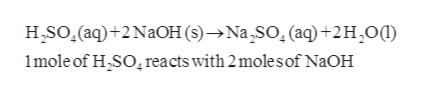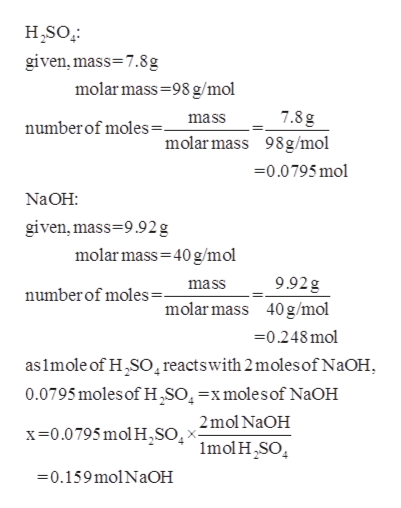Aqueous sulfuric acid (H2SO4) will react with solid sodium hydroxide (NaOH) to produce aqueous sodium sulfate (Na2SO4) and liquid water (H2O).  Suppose 7.8 g of sulfuric acid is mixed with 9.92 g of sodium hydroxide. Calculate the maximum mass of water that could be produced by the chemical reaction. Be sure your answer has the correct number of significant digits.

Question

Aqueous sulfuric acid (H2SO4) will react with solid sodium hydroxide (NaOH) to produce aqueous sodium sulfate (Na2SO4) and liquid water (H2O).  Suppose 7.8 g of sulfuric acid is mixed with 9.92 g of sodium hydroxide. Calculate the maximum mass of water that could be produced by the chemical reaction. Be sure your answer has the correct number of significant digits.

Step 1

The balanced equation for the reaction of aqueous sulfuric acid with solid sodium hydroxide to produce aqueous sodium sulfate and liquid water is given as follows:help_outlineImage TranscriptioncloseH SO.(aq)+2 NaOH (s)-Na SO. (aq)+2H20 1mole of H SO reacts with 2 molesof N2OH fullscreen
Step 2

The number of moles of sulfuric acid and ...help_outlineImage TranscriptioncloseH,SO given,mass 7.8g molar mass 98 g/mol 7.8 g numberof moles=_ mass molar mass 98g/mo 0.0795 mol Na OH: given, mass 9.92g molar mass 40 g/mol 9.92g mass numberof moles= molar mass 40g/mol -0.248mol as 1mole of H2SO reactswith 2moles of NaOH 0.0795moles of H,SO4-xmolesof NaOH x0.0795 mol H,so2mol NaOH Imol HSO 0.159molNaOH fullscreen

Want to see the full answer?

See Solution

Want to see this answer and more?

Our solutions are written by experts, many with advanced degrees, and available 24/7

See Solution
Tagged in

Quantitative analysis